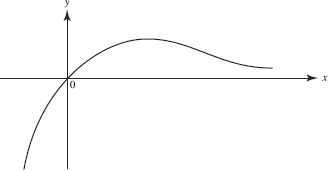# AP Calculus AB Practice Test 37

### Test Information9 questions18 minutes

Calculator Disallowed

1.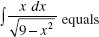2.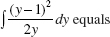3.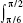cot x dx equals

4. Given f as graphed, which could be a graph of f ?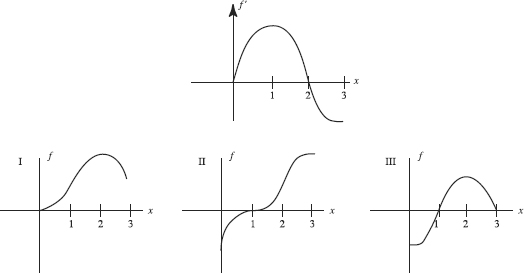5. The first woman officially timed in a marathon was Violet Piercey of Great Britainin 1926. Her record of 3:40:22 stood until 1963, mostly because of a lack of womencompetitors. Soon after, times began dropping rapidly, but lately they have been decliningat a much slower rate. Let M(t) be the curve that best represents winning marathon times in year t. Which of the following is (are) negative for t > 1963?

I. M(t)

II. M (t)

III. M (t)

6.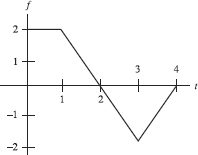The graph of f is shown above. Let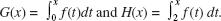Which of the following is true?

7. The minimum value of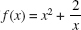on the interval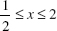is

8.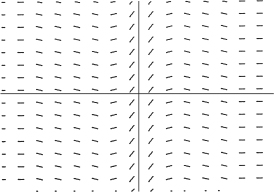Which function could be a particular solution of the differential equation whoseslope field is shown above?

9. Which of the following functions could have the graph sketched below?